# matplotlib.pyplot.subplot¶

matplotlib.pyplot.subplot(*args, **kwargs)[source]

Add a subplot to the current figure.

Wrapper of Figure.add_subplot with a difference in behavior explained in the notes section.

Call signatures:

subplot(nrows, ncols, index, **kwargs)
subplot(pos, **kwargs)
subplot(ax)

Parameters:
*args

Either a 3-digit integer or three separate integers describing the position of the subplot. If the three integers are nrows, ncols, and index in order, the subplot will take the index position on a grid with nrows rows and ncols columns. index starts at 1 in the upper left corner and increases to the right.

pos is a three digit integer, where the first digit is the number of rows, the second the number of columns, and the third the index of the subplot. i.e. fig.add_subplot(235) is the same as fig.add_subplot(2, 3, 5). Note that all integers must be less than 10 for this form to work.

projection : {None, 'aitoff', 'hammer', 'lambert', 'mollweide', 'polar', 'rectilinear', str}, optional

The projection type of the subplot (Axes). str is the name of a costum projection, see projections. The default None results in a 'rectilinear' projection.

polar : boolean, optional

If True, equivalent to projection='polar'.

sharex, sharey : Axes, optional

Share the x or y axis with sharex and/or sharey. The axis will have the same limits, ticks, and scale as the axis of the shared axes.

label : str

A label for the returned axes.

Returns:
axes : an axes.SubplotBase subclass of Axes (or a subclass of Axes)

The axes of the subplot. The returned axes base class depends on the projection used. It is Axes if rectilinear projection are used and projections.polar.PolarAxes if polar projection are used. The returned axes is then a subplot subclass of the base class.

Other Parameters:
**kwargs

This method also takes the keyword arguments for the returned axes base class. The keyword arguments for the rectilinear base class Axes can be found in the following table but there might also be other keyword arguments if another projection is used.

Property Description
adjustable {'box', 'datalim'}
agg_filter a filter function, which takes a (m, n, 3) float array and a dpi value, and returns a (m, n, 3) array
alpha float
anchor 2-tuple of floats or {'C', 'SW', 'S', 'SE', ...}
animated bool
aspect {'auto', 'equal'} or num
autoscale_on bool
autoscalex_on bool
autoscaley_on bool
axes_locator Callable[[Axes, Renderer], Bbox]
axisbelow bool or 'line'
clip_box Bbox
clip_on bool
clip_path [(Path, Transform) | Patch | None]
contains callable
facecolor color
fc color
figure Figure
frame_on bool
gid str
in_layout bool
label object
navigate bool
navigate_mode unknown
path_effects AbstractPathEffect
picker None or bool or float or callable
position [left, bottom, width, height] or Bbox
rasterization_zorder float or None
rasterized bool or None
sketch_params (scale: float, length: float, randomness: float)
snap bool or None
title str
transform Transform
url str
visible bool
xbound unknown
xlabel str
xlim (left: float, right: float)
xmargin float greater than -0.5
xscale {"linear", "log", "symlog", "logit", ...}
xticklabels List[str]
xticks list
ybound unknown
ylabel str
ylim (bottom: float, top: float)
ymargin float greater than -0.5
yscale {"linear", "log", "symlog", "logit", ...}
yticklabels List[str]
yticks list
zorder float

Notes

Creating a subplot will delete any pre-existing subplot that overlaps with it beyond sharing a boundary:

import matplotlib.pyplot as plt
# plot a line, implicitly creating a subplot(111)
plt.plot([1,2,3])
# now create a subplot which represents the top plot of a grid
# with 2 rows and 1 column. Since this subplot will overlap the
# first, the plot (and its axes) previously created, will be removed
plt.subplot(211)


If you do not want this behavior, use the Figure.add_subplot method or the pyplot.axes function instead.

If the figure already has a subplot with key (args, kwargs) then it will simply make that subplot current and return it. This behavior is deprecated. Meanwhile, if you do not want this behavior (i.e., you want to force the creation of a new suplot), you must use a unique set of args and kwargs. The axes label attribute has been exposed for this purpose: if you want two subplots that are otherwise identical to be added to the figure, make sure you give them unique labels.

In rare circumstances, add_subplot may be called with a single argument, a subplot axes instance already created in the present figure but not in the figure's list of axes.

Examples

plt.subplot(221)

# equivalent but more general
ax1=plt.subplot(2, 2, 1)

# add a subplot with no frame
ax2=plt.subplot(222, frameon=False)

plt.subplot(223, projection='polar')

# add a red subplot that shares the x-axis with ax1
plt.subplot(224, sharex=ax1, facecolor='red')

#delete ax2 from the figure
plt.delaxes(ax2)

#add ax2 to the figure again
plt.subplot(ax2)


## Examples using matplotlib.pyplot.subplot¶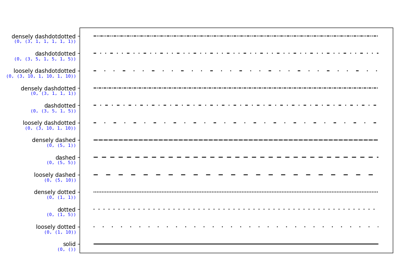Linestyles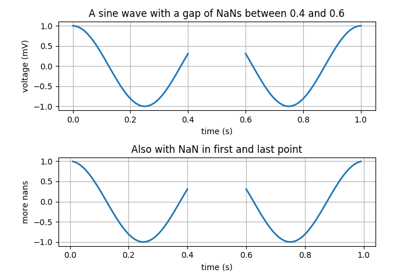Nan Test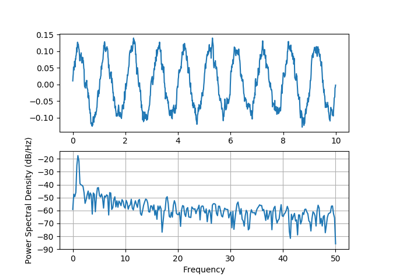Psd Demo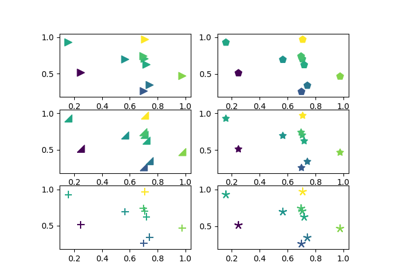Scatter Star Poly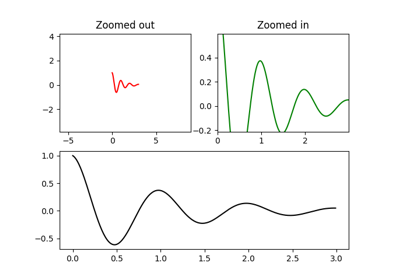Zooming in and out using Axes.margins and the subject of "stickiness"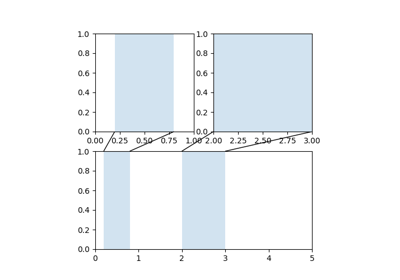Axes Zoom Effect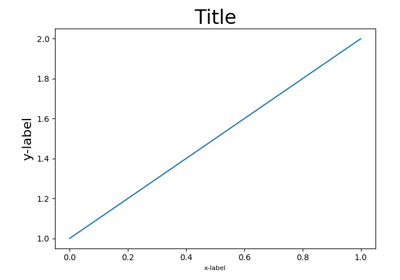Resizing axes with tight layout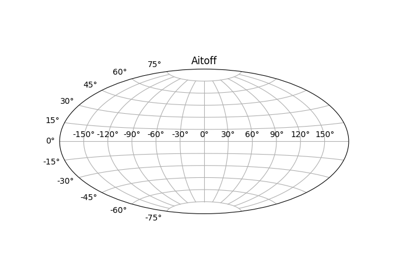Geographic Projections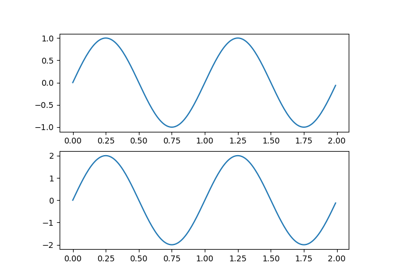Multiple Figs Demo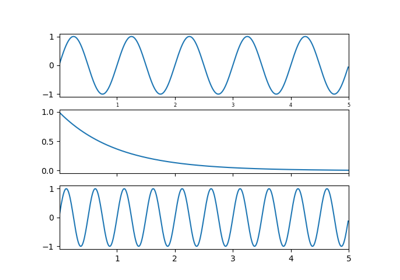Shared Axis Demo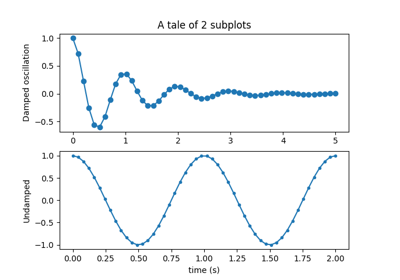Multiple subplots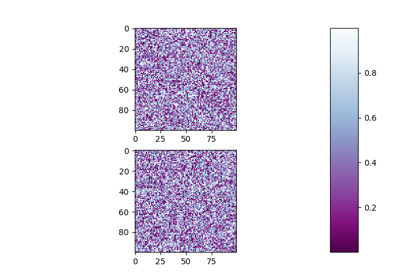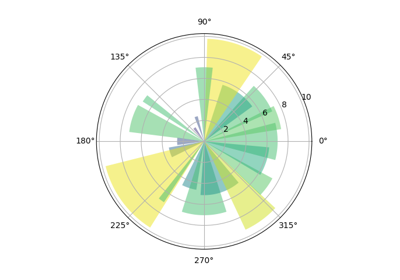Pie chart on polar axis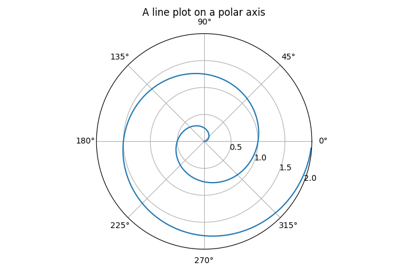Polar Demo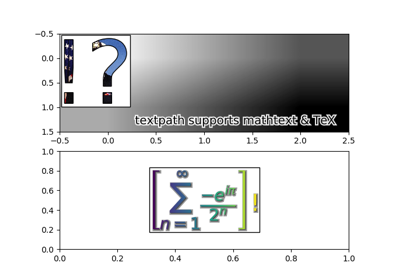Demo Text Path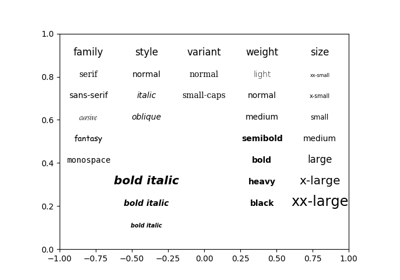Fonts demo (object-oriented style)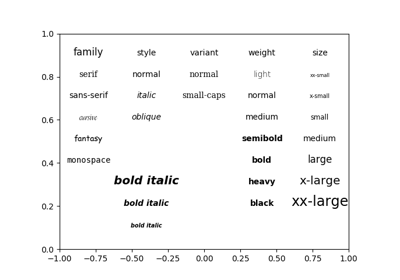Fonts demo (kwargs)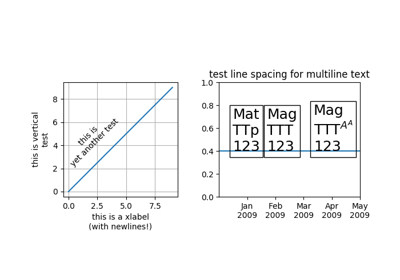Multiline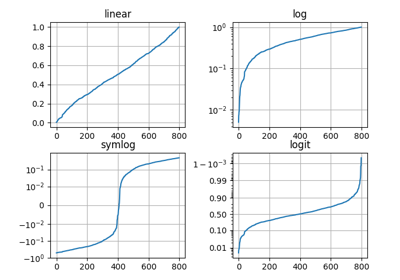Pyplot Scales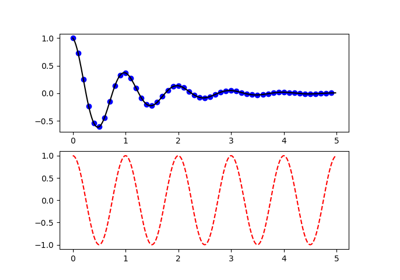Pyplot Two Subplots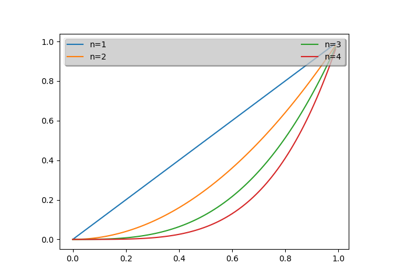Whats New 0.98.4 Legend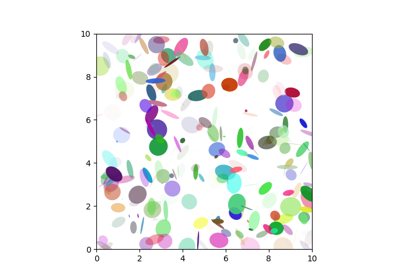Ellipse Demo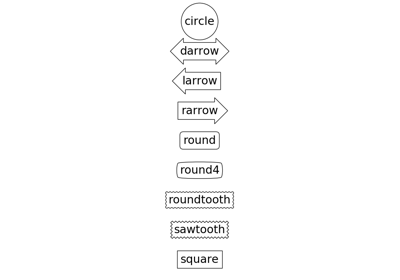Fancybox Demo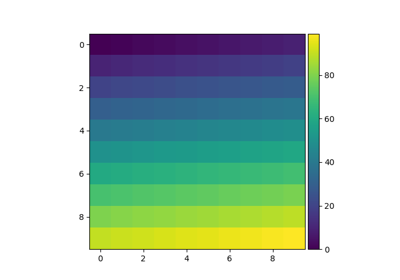Simple Colorbar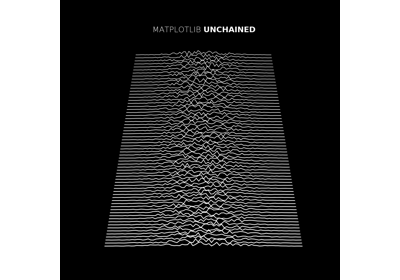MATPLOTLIB UNCHAINED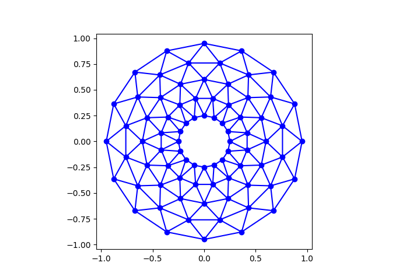Trifinder Event Demo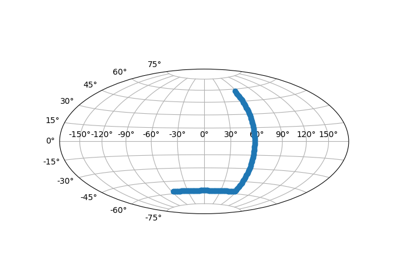Custom projection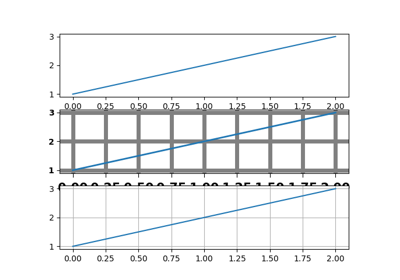Customize Rc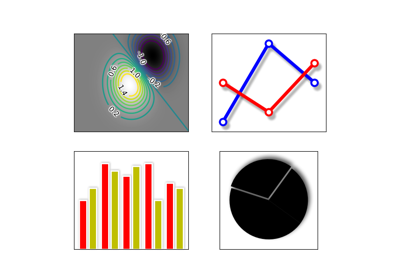Demo Agg Filter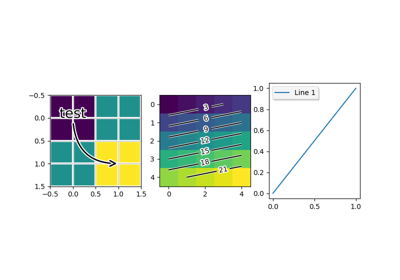Patheffect Demo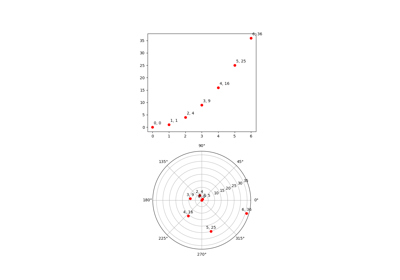Transoffset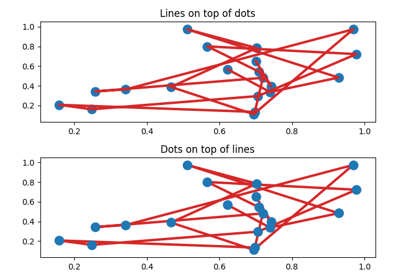Zorder Demo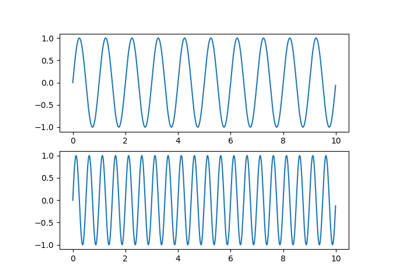Sharing axis limits and views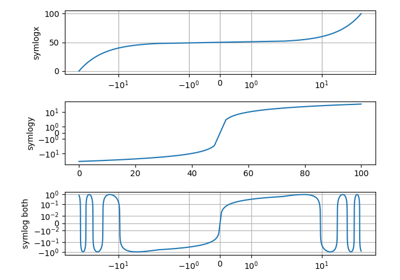Symlog Demo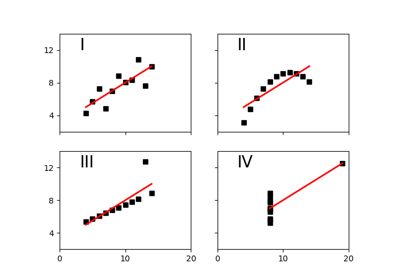Anscombe's Quartet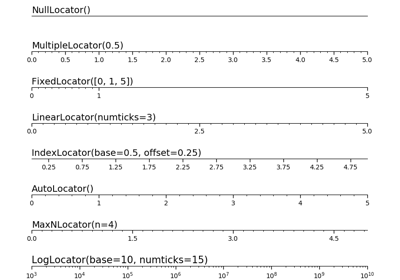Tick locators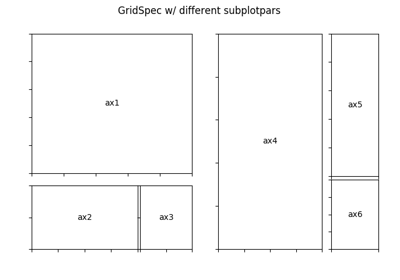Demo Gridspec03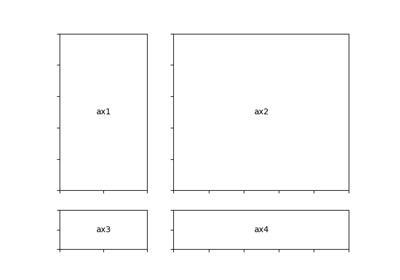Demo Gridspec05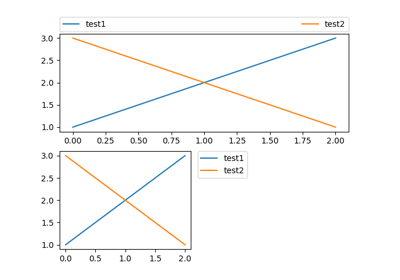Simple Legend01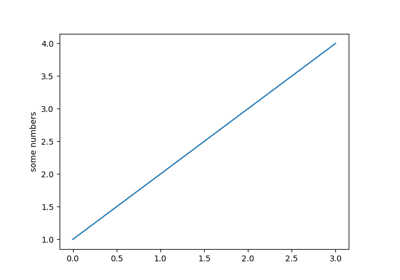Pyplot tutorial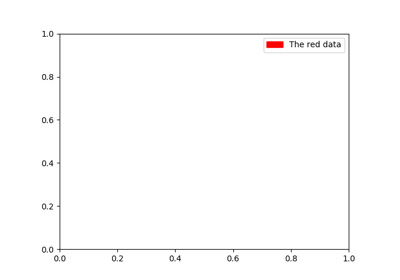Legend guide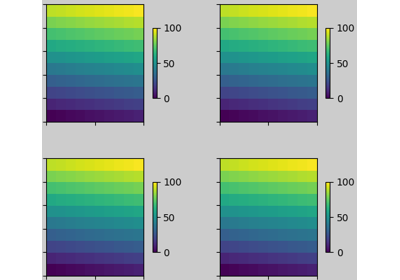Constrained Layout Guide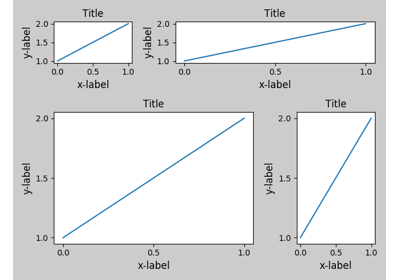Tight Layout guide